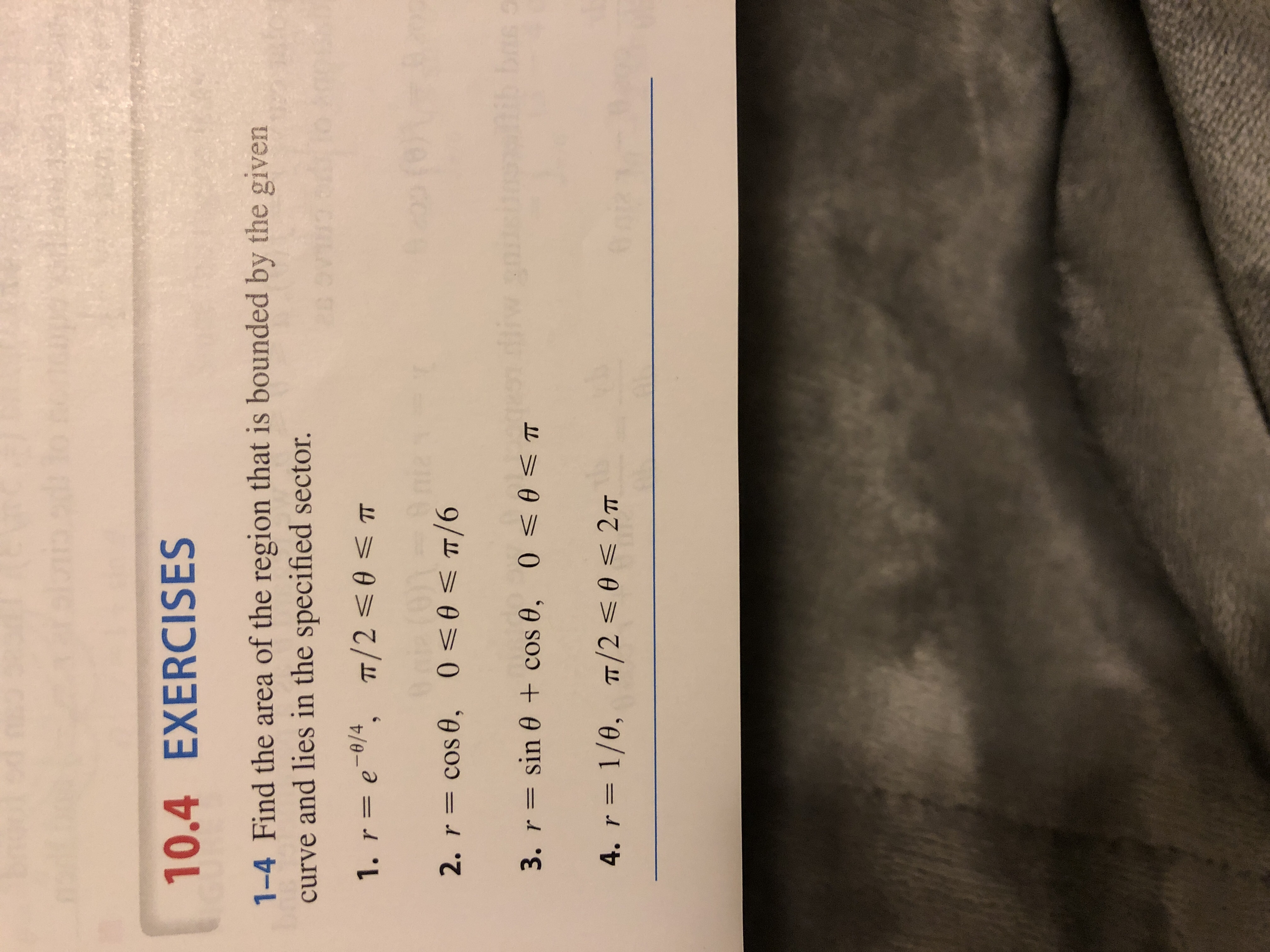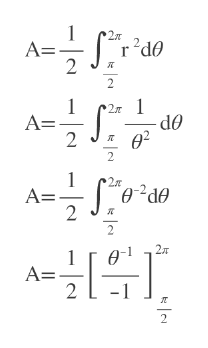# 10.4 EXERCISES1-4 Find the area of the region that is bounded by the givencurve and lies in the specified sector.1. r = e 0/4, TT/2 0T2. r cos 0, 00T/6i6us s3. r= sin 0 + cos 0, 0

Question
3 views

Find the area of the region that is bounded by the given curve and lies in the specified sector.

4. r = 1/θ,    (pi/2) <= θ <= 2pihelp_outlineImage Transcriptionclose10.4 EXERCISES 1-4 Find the area of the region that is bounded by the given curve and lies in the specified sector. 1. r = e 0/4, TT/2 0T 2. r cos 0, 00T/6 i6 us s 3. r= sin 0 + cos 0, 0 <0 < T 4. r 1/0, T 2 02T 6 fullscreen
check_circle

Step 1

We have to use area formula of polar coordinates with a= pi/2 and b=2pi

Step 2

We plug r=1/theta in the formula and then try to integ...help_outlineImage TranscriptioncloseS 2 A= 2 r 1 A= 2 2 1 OP 2 2 A= 2 2T A= - 2 fullscreen

### Want to see the full answer?

See Solution

#### Want to see this answer and more?

Solutions are written by subject experts who are available 24/7. Questions are typically answered within 1 hour.*

See Solution
*Response times may vary by subject and question.
Tagged in

### Other